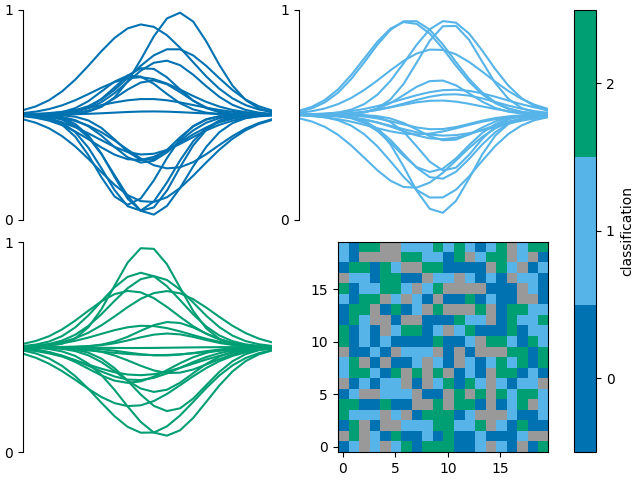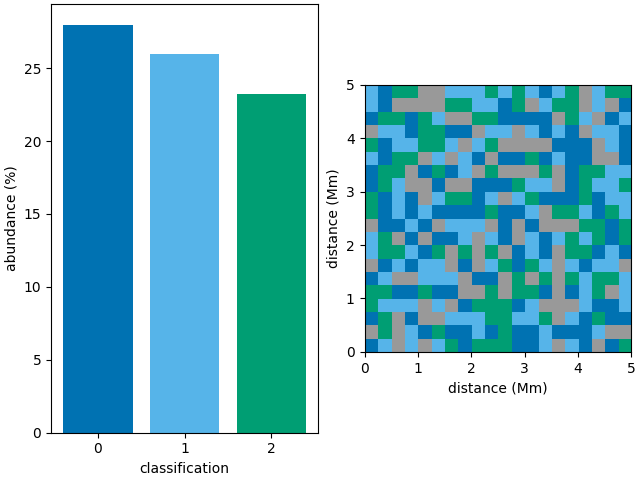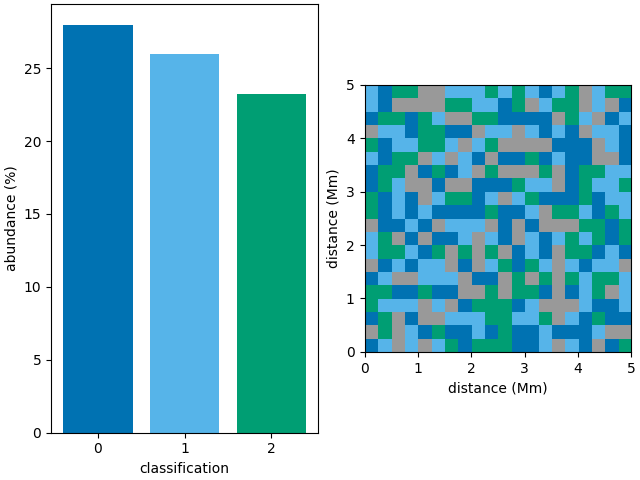# Combine multiple classification plots

This is an example showing how to use multiple classification plotting functions in a single figure.

First we shall create a random 3D grid of classifications that can be plotted. Usually you would use a method such as `mcalf.models.ModelBase.classify_spectra()` to classify an array of spectra.

```from mcalf.tests.helpers import class_map as c

t = 1  # One image
x = 20  # 20 coordinates along x-axis
y = 20  # 20 coordinates along y-axis
n = 3  # Possible classifications [0, 1, 2]

class_map = c(t, x, y, n)  # 3D array of classifications (t, y, x)
```

Next we shall create a random array of spectra each labelled with a random classifications. Usually you would provide your own set of hand labelled spectra taken from spectral imaging observations of the Sun. Or you could provide a set of spectra labelled by the classifier.

```from mcalf.tests.visualisation.test_classifications import spectra as s

n = 400  # 200 spectra
w = 20  # 20 wavelength points for each spectrum
low, high = 0, 3  # Possible classifications [0, 1, 2]

# 2D array of spectra (n, w), 1D array of labels (n,)
spectra, labels = s(n, w, low, high)
```

If a GridSpec returned by the plot_classification function has free space, a new axes can be added to the returned GridSpec. We can then request plot_class_map to plot onto this new axes. The colorbar axes can be set to `fig.axes` such that the colorbar takes the full height of the figure, as in this case, its colours are the same as the line plots.

```import matplotlib.pyplot as plt
from mcalf.visualisation import plot_classifications, plot_class_map

fig = plt.figure(constrained_layout=True)

gs = plot_classifications(spectra, labels, nrows=2, show_labels=False)

plot_class_map(class_map, ax=ax, colorbar_settings={
'ax': fig.axes,
'label': 'classification',
})
``````<matplotlib.image.AxesImage object at 0x7f7b3fde0a00>
```

The function `mcalf.visualisation.init_class_data`()` is intended to be an internal function for generating data that is common to multiple plotting functions. However, it may be used externally if necessary.

```from mcalf.visualisation import init_class_data, bar

fig, ax = plt.subplots(1, 2, constrained_layout=True)

data = init_class_data(class_map, resolution=(0.25, 0.25), ax=ax)

bar(data=data, ax=ax)
plot_class_map(data=data, ax=ax, show_colorbar=False)
``````<matplotlib.image.AxesImage object at 0x7f7b37f09160>
```

The following example should be equivalent to the example above,

```fig, ax = plt.subplots(1, 2, constrained_layout=True)

bar(class_map, ax=ax)
plot_class_map(class_map, ax=ax, show_colorbar=False,
resolution=(0.25, 0.25))
``````<matplotlib.image.AxesImage object at 0x7f7b5ecd6610>
```

Total running time of the script: ( 0 minutes 1.136 seconds)

Gallery generated by Sphinx-Gallery